International
Tables for
Crystallography
Volume B
Reciprocal space
Edited by U. Shmueli

International Tables for Crystallography (2010). Vol. B, ch. 1.3, p. 46   | 1 | 2 |

## Section 1.3.2.6.10.1. Classicaltheory

G. Bricognea

aGlobal Phasing Ltd, Sheraton House, Suites 14–16, Castle Park, Cambridge CB3 0AX, England, and LURE, Bâtiment 209D, Université Paris-Sud, 91405 Orsay, France

#### 1.3.2.6.10.1. Classicaltheory

| top | pdf |

The spaceconsists of (equivalence classes of) complex-valued functions f on the circle which are summable, i.e. for which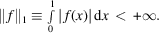It is a convolution algebra: If f and g are in, thenis in.

The mth Fourier coefficient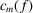of f,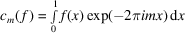is bounded: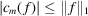, and by the Riemann–Lebesgue lemma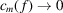as. By the convolution theorem,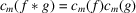.

The pth partial sum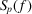of the Fourier series of f,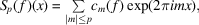may be written, by virtue of the convolution theorem, as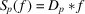, whereis the Dirichlet kernel. Becausecomprises numerous slowly decaying oscillations, both positive and negative,may not converge towards f in a strong sense as. Indeed, spectacular pathologies are known to exist where the partial sums, examined pointwise, diverge everywhere (Zygmund, 1959, Chapter VIII). When f is piecewise continuous, but presents isolated jumps, convergence near these jumps is marred by the Gibbs phenomenon:always overshoots the mark' by about 9%, the area under the spurious peak tending to 0 asbut not its height [see Larmor (1934)for the history of this phenomenon].

By contrast, the arithmetic mean of the partial sums, also called the pth Cesàro sum,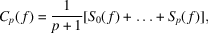converges to f in the sense of thenorm: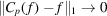as. If furthermore f is continuous, then the convergence is uniform, i.e. the error is bounded everywhere by a quantity which goes to 0 as. It may be shown that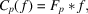whereis the Fejér kernel.has overthe advantage of being everywhere positive, so that the Cesàro sums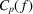of a positive function f are always positive.

The de la Vallée Poussin kernelhas a trapezoidal distribution of coefficients and is such thatif; thereforeis a trigonometric polynomial with the same Fourier coefficients as f over that range of values of m.

The Poisson kernelwith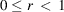gives rise to an Abel summation procedure [Tolstov (1962, p. 162); Whittaker & Watson (1927, p. 57)] since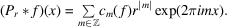Compared with the other kernels,has the disadvantage of not being a trigonometric polynomial; however,is the real part of the Cauchy kernel (Cartan, 1961; Ahlfors, 1966):and hence provides a link between trigonometric series and analytic functions of a complex variable.

Other methods of summation involve forming a moving average of f by convolution with other sequences of functionsbesidesofwhich tend towards δ' as. The convolution is performed by multiplying the Fourier coefficients of f by those of, so that one forms the quantities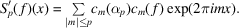For instance the sigma factors' of Lanczos (Lanczos, 1966, p. 65), defined bylead to a summation procedure whose behaviour is intermediate between those using the Dirichlet and the Fejér kernels; it corresponds to forming a moving average of f by convolution withwhich is itself the convolution of a rectangular pulse' of widthand of the Dirichlet kernel of order p.

A review of the summation problem in crystallography is given in Section 1.3.4.2.1.3.

### References

Ahlfors, L. V. (1966). Complex Analysis. New York: McGraw-Hill.
Cartan, H. (1961). Théorie des Fonctions Analytiques. Paris: Hermann.
Lanczos, C. (1966). Discourse on Fourier Series. Edinburgh: Oliver & Boyd.
Larmor, J. (1934). The Fourier discontinuities: a chapter in historical integral calculus. Philos. Mag. 17, 668–678.
Tolstov, G. P. (1962). Fourier Series. Englewood Cliffs: Prentice-Hall.
Whittaker, E. T. & Watson, G. N. (1927). A Course of Modern Analysis, 4th ed. Cambridge University Press.
Zygmund, A. (1959). Trigonometric Series, Vols. 1 and 2. Cambridge University Press.